# Hedge and Bandits

## 1 Introduction

We will prepare ourselves for a non-convex setting that uses results for OCO.

• First derive Hedge as a particular case of FTRL.
• Introduce the Bandit setting for which Hedge can almost be immediately applied.

## 2 Expert problem and Hedge

##### Problem formulation

In the expert problem we are to select a weighing of experts which minimize a loss that is simply the sum of losses associated with each expert. An example of this setting could be portfolio selection where each stock is an expert and the loss is the negative return. Formalized in our OCO framework this corresponds to a decision space being the finite dimensional simplex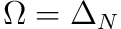and the loss linear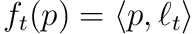for which we will further assume boundedness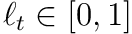.

1. Player picks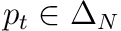.
2. Player observed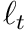and suffers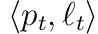.

We are thus interested in minimizes the following loss,with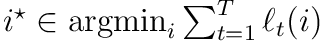.

### 2.2 FTRL

If we want to apply FTRL on this we need to choose the regularizer. A natural choice when the decision set is the simplex is to choose the (negative) entropyOur proposed solution is thus,

FTRL with negative entropic regularization:### 2.3 Regret Bounds

For the regret bound to go through we need to prove that the negative entropy function is strongly convex w.r.t. the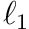-norm,Under the-norm this reduces to the well-known Pinsker’s inequality which relates the total variation distance (or half the-norm) with the KL-divergenceWe need the deriatives of negative entropy: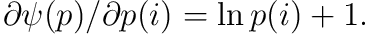,Knowing that our assumptions holds we can move on to simplifying the general regret bound obtained with FTRL for our particular case. Recall the bound,We need to bound the dual norm of gradient and the term depending on the negative entropy. For the former term we can bound it through the loss,The sum of squares of these terms are thus trivially bounded by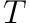.

Concerning the negative entropy: the discrete distribution which maximizes the negative entropy is a distribution with point mass while the minimum is a uniform distribution. The negative entropy for these two distributions are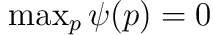and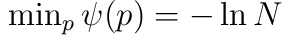respectively. Thus we obtain the following boundBe aware that this can be tightened to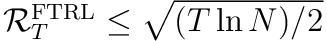. See e.g. .

,#### 2.3.1 Regret bound for samples

Say we are incurring loss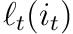where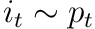instead of incurring the loss over the entire mixed strategy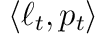. In expectation these are obviously the same,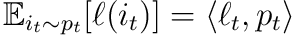. So the following regretsare equivalent in expectation,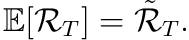However, we might be interested in bounded the regret with high probability insteadThere is a good discussion on high probability regret bounds mainly in the context of Bandits which we will cover promptly. The motivation is simple: even though we might be doing well in expectation the variance might be very high. So our algorithm is still very risky in the sense that it is likely that a concrete instantiation will have high regret.

. This can naturally be reduced to a concentration argument where we make a statement about how far most of the random variables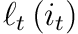are from the expectation.

Subtlety: the history, that the environment may depend on, is thus now a random variable. We call the adversary oblivious if it chooses the stream of losses prior to the game, i.e. the randomness of our algorithm does not effect what environment we are compared against. This is the setting we will be considering.

Even in this simplifying setting be can still ask to bound the regret with high probability instead of the expectation. We will use a general concentration inequality which relies on a martingale difference sequences.

(Martingale difference sequence)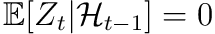where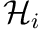is the filtration.

(Azuma-Hoeffding inequality) If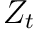is martingale difference sequence thenwith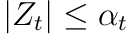.

This readily lets us bound the regret. Pick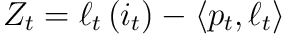. We then know that

•form a martingale difference sequence since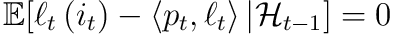.

•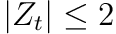by boundedness assumption on the loss.

So we can apply the Azuma-Hoeffding inequality. With probability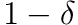we haveThis gives us a high probability regret bound.

(High probability Regret Bound) With probability at leastand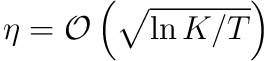,### 2.4 Reducing the algorithm to Softmax

At each step we need to solve the constraint minimization problem,This turns out to have a closed form solution for our choice of regularization.

To obtain this, notice that the constraint is an equality constraint,So we can obtain the exact solution by instead considering the Langrange function,Setting the derivatives to zeroTo specify the remaining unknown,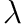, we use its associated first order condition and plug-in our derived expressions for the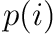’s,This gives us an update rule as follows:

(Hedge) It updates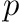element-wise as follows,#### 2.4.1 Re-reformulation: multiplicative weight update

Alternatively we can rewrite this to save recomputation by reusing the previously computed weights.

(Multiplicative Weight Update variant of Hedge) It updateselement-wise as follows,### 2.5 Final Remarks

So to summarize: for the simplex introducing the entropy as regularizer simply reduces to replacing the max (if we were to use Follow The Leader) with a softmax.

This method goes by many other names in the literature apart from Hedge: Exponentiated Gradient Descent, Weighted Majority and Multiplicative Weight Update to mention the most common.

### 2.6 Exercises:

• What happens if we choose a different norm than?
• What about a different regularizer than negative entropy?

## 3 Multi-Armed Bandit

In multi-armed bandit we consider a slight modification to the setting for which we applied Hedge. The difference lies in considering limited feedback. That is, we only observe the losswhich we incur instead of the entire function.

(Bandit setting) The player is given a fixed decision set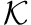. At each round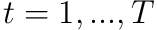:

1. Player pick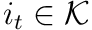.
2. The environment picks a convex loss vector.
3. The player then observes and suffers loss.
##### Exploration/Exploitation

Central to this setting is the exploration-exploitation tradeoff: we want to learn the best arm to pull (explore) but also pull the right arm (exploit). It is a tradeoff since learning something about a particular arm now requires us to choose it and incur the associated loss.

##### Finite arms

Much of the notation will allow a continuos(i.e. infinite arms) but we will only consider finite arm bandits in this post, i.e. when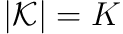.

##### Applying Hedge

We cannot naively apply Hedge as it would require full information of the previous losses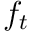. What we can do is construct an unbiased estimator of the gradient on which we then apply Hedge.

(Importance-Weighted Estimator) Say you sample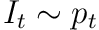and constructthen this estimator is unbiasedCombining importance-weighting with Hedge leads to what has been called Exp3.

(Exp3)
Initialize uniformly: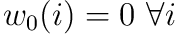Then for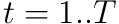##### Regret analysis

The standard FTRL analysis would fail. To see why, let’s look at the regret on our estimatorIn order to say anything on the actual regret we need to take the expectation,Notice how it will blow up if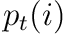is very small for some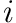The initial analysis bounded the regret by explicitly exploring uniformly based on a coin flip. This waywas ensured to be sufficiently big for all.

.

We can tighten the analysis, however, using some tricks almost exclusively reserved for the particular case of Exp3.

(Exp3 Regret bound)proof can be found here.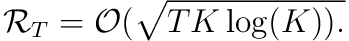Remarks:

• The lower bound for adversarial regret is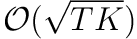See e.g. this proof.

. So we achieve minimax regret up to a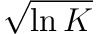factor.
• Compared with the full information setting we are competitive up to a factor of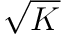.
• Francesco Orabona has a very different take on the proof coming from an Online Convex Optimization perspective that might be of interest.

## 4 Final Remarks

This presentation primarily pulls from the following sources:

• The Hedge part heavily relies on Haipeng’s lecture note 3 except for the (standard) derivation with Langrange multiplier which I weirdly enough didn’t come across anywhere.
• The Bandit part is loosly based on Haipeng’s lecture note 12 and lecture note 8 but fundamentally altered to prepare us for Gaussian Processes with Multiplicative Weights which we will cover next.

I’ve skipped through some standard results in the Bandit literature: Explore-then-exploit and Upper Confidence Bound (UCB) to not get us too afar astray from the analysis we have seen so far in OCO. A good starting point for the curious reader is either Haipeng’s notes or one of the many books on Bandits (the literature is vast!).

 N. Cesa-Bianchi and G. Lugosi, Prediction, learning, and games. Cambridge university press, 2006.